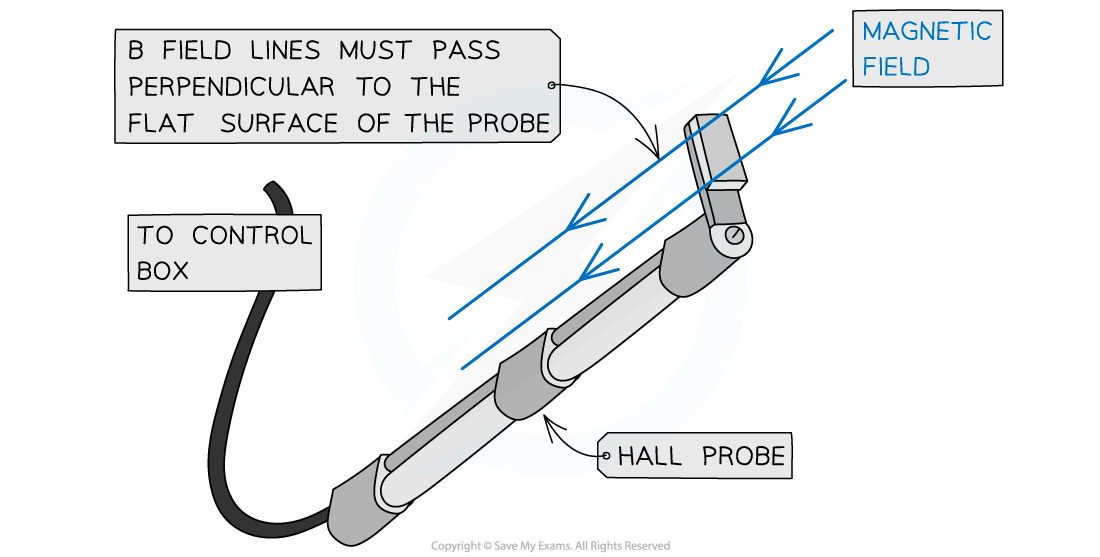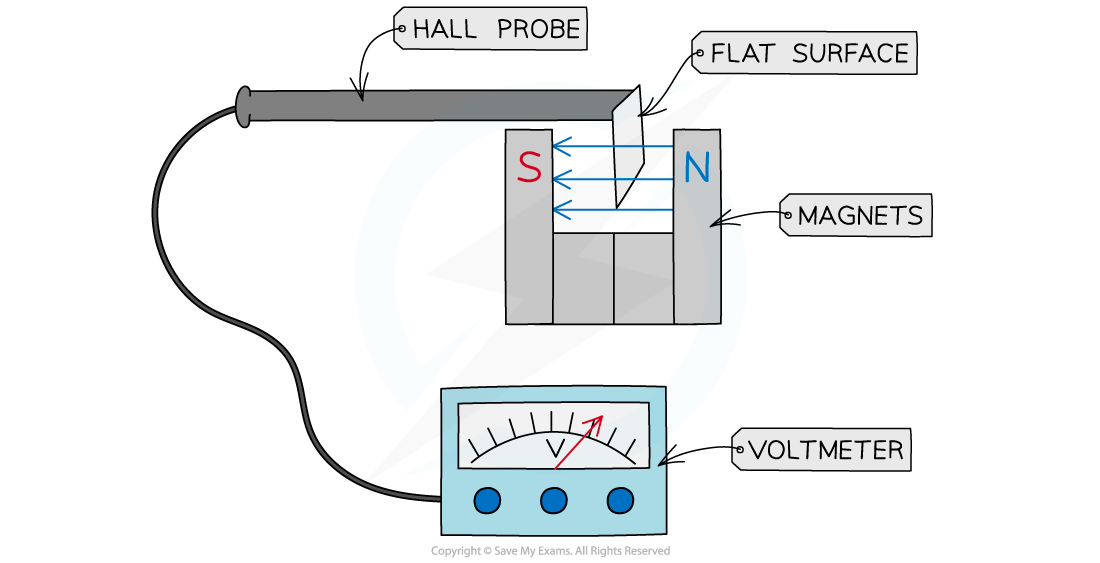# CIE A Level Physics复习笔记20.1.7 Using a Hall Probe

### Measuring Magnetic Flux Density using a Hall Probe

• A Hall probe can be used to measure the magnetic flux density between two magnets based on the Hall effect
• It consists of a cylinder with a flat surface at the endA Hall probe consists of a flat surface and is held so the magnetic field lines are perpendicular to it

• To measure the magnetic flux density between two magnets, the flat surface of the probe must be directed between the magnets so the magnetic field lines pass completely perpendicular to this surface• The probe is connected to a voltmeter to measure the Hall voltage
• If the probe is not held in the correct orientation (perpendicular to the field lines), the voltmeter reading will be reduced
• Since the Hall voltage is directly proportional to the magnetic flux density, the flux density of the magnets can be obtained
• A Hall probe is sensitive enough to measure even the Earth’s magnetic flux density

#### Worked Example

A Hall probe is placed near one end of a solenoid, as shown in the diagram.The Hall probe is rotated about the axis XY.State and explain why the magnitude of the Hall voltage varies.

• The Hall voltage depends on angle between the magnetic field and the plane of the probe
• The Hall voltage reaches a maximum when the field is perpendicular to the probe
• The Hall voltage is zero when the field is parallel to the probe